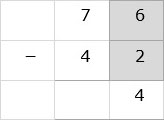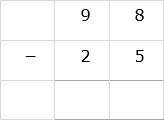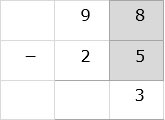# Subtraction of Two 2-digit Numbers Without Borrowing

Subtract the numbers 76 − 42

### Solution

Step 1:

We line up the numbers vertically.Step 2:

Here, subtract the numbers in the ones column first. 6 – 2 = 4. Write 4 below ones column.Step 3:

Next subtract the numbers in the tens column. 7 – 4 = 3. Write down 3 below tens column. So the final answer is 34.Subtract 25 from 98

### Solution

Step 1:

Align the numbers vertically.Step 2:

Here, subtract the numbers in the ones column first. 8 – 5 = 3. Write down 3 below ones column.Step 3:

Next the numbers in the tens column are subtracted. 9 – 2 = 7. Write 7 below tens column. So the final answer is 73.